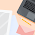How to implement Least Recently Used(LRU) cache algorithm code (AMCAT automata code)

Here is the Least Recently Used(LRU) cache algorithm code written in C++. This question is asked in Amcat Automata. Lets see the question first. We have seen all the important question of AMCAT. See here.

Here is the Least Recently Used(LRU) cache algorithm code written in C++. This question is asked in Amcat Automata. Lets see the question first. We have seen all the important question of AMCAT here.

Question: The LeastRecentlyUsed(LRU) cache algorithm exists the element from the cache(when it's full) that was leastrecentlyused.

After an element is requested from the cache, it should be added to the cache(if not already there) and considered the mostrecentlyused element in the cache.

Given the maximum size of the cache and a list of integers(to request from the cache), calculate the number of cache misses using the LRU cache algorithm. A cache miss occur when the requested integer does not exist in the cache.

Initially, the cache is empty. The input to the function LruCountMiss shall consist of an integer max_cache_size, an array pages and its length len.

The function should return an integer for the number of cache misses using the LRU cache algorithm. Assume that the array pages always has pages numbered from 1 to 50.
TESTCASES:
TESTCASE1:
INPUT:
3,[7,0,1,2,0,3,0,4,2,3,0,3,2,1,2,0],16
EXPECTED RETURN VALUE:
11
TESTCASE 2:
INPUT:
2,[2,3,1,3,2,1,4,3,2],9
EXPECTED RETURN VALUE:
8
EXPLANATION:
The following page numbers are missed one after the other 2,3,1,2,1,4,3,2.This results in 8 page misses.
Sample CODE:
int lruCountMiss(int max_cache_size, int *pages,int len)
{/
/write tour code
}
CODE in C++
``` #include<iostream>
using namespace std;

int lruCountMiss(int max_cache_size, int *pages,int len)
{
int miss=0,cache[max_cache_size]; //variable miss and cache declared
for (int i=0;i<max_cache_size;i++){ /*this is for clearing value of cache i.e. to empty cache. I used any value here*/
cache[i]=0x87787;
}

for (int i=0;i<len;i++){ //for loop for all the value from list one by one
for(int j=0;j<max_cache_size;j++){ //loop to check the cache value, if it matches the value
if (pages[i]==cache[j]){
for (int k=i; k<max_cache_size;k++){ /*if the value is already present in the cache then shifting values from the present value.*/
cache[i]=cache[i+1];
}
cache[max_cache_size-1]=pages[i]; //updating the last value of cache

break;
}
else if(j==(max_cache_size-1)){
for (int l=0; l<max_cache_size;l++){ /*if the value is not present in the cache then shifting values from starting.*/
cache[l]=cache[l+1];
}
cache[max_cache_size-1]=pages[i];//updating the last value of cache
miss++;

}
}}
return miss;}//returning the Miss

int main(){ //main function
cout<< "This is the Least Recently Used(LRU) cache algorithmfrom mindxmaster.com\n";
cout<< "We are checking two cases.\n"<<"Case 1:t"<<"Array values are [7,0,1,2,0,3,0,4,2,3,0,3,2,1,2,0] nand cache size= 3; and total values to check are 16: n We got the miss. ";
int days,pages[]={7,0,1,2,0,3,0,4,2,3,0,3,2,1,2,0}; //array to pass through function
int *cellsptr=pages; //creating array values to pointer
cout<<"Miss =t"<<lruCountMiss(3,cellsptr,16);//passing to function
cout<<"nnCase 2:t"<<"Array values are [2,3,1,3,2,1,4,3,2] nand cache size= 2; and total values to check are 9: n We got the miss. ";
int pages2[]={2,3,1,3,2,1,4,3,2};
int *cellsptr2=pages2; //creating array values to pointer
cout<<"Miss =t"<<lruCountMiss(2,cellsptr2,9);//passing to function
return 0;
}```Least recently used cache algorithmBLOGGER: 2
1.thanks for the solution but i think following places should hold "j" instead of "i"

if (pages[i]==cache[j]){
for (int k=/*j*/i; k<max_cache_size;k++){ /*if the value is already present in the cache then shifting values from the present value.*/
cache[/*j*/i]=cache[/*j*/i+1];
}

1.Ok, I will clear the logic and update, Thanks for telling.

## Related Articles

Name

ltr
item
MindxMaster: How to implement Least Recently Used(LRU) cache algorithm code (AMCAT automata code)
How to implement Least Recently Used(LRU) cache algorithm code (AMCAT automata code)
Here is the Least Recently Used(LRU) cache algorithm code written in C++. This question is asked in Amcat Automata. Lets see the question first. We have seen all the important question of AMCAT. See here.
https://1.bp.blogspot.com/-RJzmumerMgc/Vlq8q3UuCZI/AAAAAAAAA5w/MaQVgY9OvtA/s1600/LRU.jpg
https://1.bp.blogspot.com/-RJzmumerMgc/Vlq8q3UuCZI/AAAAAAAAA5w/MaQVgY9OvtA/s72-c/LRU.jpg
MindxMaster
https://www.mindxmaster.com/2015/11/how-to-implement-least-recently-usedlru.html
https://www.mindxmaster.com/
https://www.mindxmaster.com/
https://www.mindxmaster.com/2015/11/how-to-implement-least-recently-usedlru.html
true
5332415103371288268
UTF-8